Latest Banking jobs   »

# Reasoning Ability Quiz For SBI Clerk Prelims 2022- 21st September

Directions (1-4): Study the information carefully and answer the questions given below.
Point A is 10m south of point E. Point D is 10m north of point I. Point G is 8m west of point I. Point F is 20m north of point G. Point A is 4m east of point H. Point C is 16m north of point H. Point B is 12m west of point D. Point F is east of point C and the distance between them is half of the distance between Point H and Point A.

Q1. What is the shortest distance between B and E?
(a) 18m
(b) √52m
(c) √50m
(d) 21m
(e) None of these

Q2. Point G is in which direction with respect to C?
(a) North-East
(b) South-East
(c) East
(d) North-West
(e) South-West

Q3. Four of the following five are alike in certain way based from a group, find the one that does not belong to that group?
(a) A-D
(b) B-C
(c) G-D
(d) I-A
(e) H-D

Q4. If point S is 11m south of point F then in which direction of Point S with respect to point D?
(a) West
(b) South-west
(c) North
(d) East
(e) North-east

Q5. Mohan started walking from point A to reach point B, which is 10 km east of A. Point C is 4 km south to B. Point E is 2 km east of F, which is 6km north to D. Point D is 5km to east of C. What is the shortest distance between point E and point C?
(a)20
(b)10
(c)√75
(d)√85
(e)None of these

Direction (6-7): Read the information carefully and answer the question:
Point M is 7m south of point Q. Point F is 7m east of point I. Point H is 5m north of point N. Point L is 12m south of point I. Point N is 4m east of point L. Point Q is 6m east of point H.

Q6. If point X is 3m west of Point F, then what is the shortest distance between point X and H?
(a) 7m
(b) 9m
(c) 6m
(d) 8m
(e) None-of-these

Q7. In which direction point N with respect to point M?
(a) East
(b) North
(c) South east
(d) West
(e) North west

Directions (8-10): Study the following information carefully and answer the given questions.
Point A is 18m north of point B. Point E is 7m south of point F. Point B is 20m west of point C which is 8m south of point D. Point G is 10m west of point F and Point D is 15m east of point E.

Q8. What is the direction of point B with respect to point F?
(a) East
(b) South-west
(c) South
(d) North-east
(e) None of these

Q9. What is the shortest distance between point G and Point A?
(a) 4m
(b) 12m
(c) √34m
(d)√51m
(e) None of these

Q10. What is the direction of point E with respect to point C?
(a) West
(b) North
(c) South-east
(d) North-west
(e) None of these

Directions (11-12): Study the following information to answer the given questions.
Point Q is 15m east of point P. Point U is 6m south of point V. Point R is 18m west of point S. Point Q is 12m north of point R. Point S is 6m south of point T, which is 8m east of point U.

Q11. What is the direction of point P with respect to point T?
(a) South-West
(b) North-East
(c) North-West
(d) South-East
(e) None of these

Q12. What is the shortest distance between point U to point R?
(a) 8m
(b) 7m
(c) 12m
(d) 10m
(e) None of these

Direction (13-15): Study the given information and answer the following question.
Point A is 13m west of point B. Point D is 4m south of point B. Point C is east of 9m of point M. Point T is 12m west of point D. Point Y is 16m north of point C. Point Y is 2m west of point A.

Q13. What is the shortest distance between Y and T?
(a)√18m
(b) 5m
(c) 7m
(d) 2m
(e) None of these

Q14. Point Y is in which direction with respect to D?
(a) North-East
(b) East
(c) West
(d) North-West
(e) None of these

Q15. If Point K is 3m east of point C then what is the shortest distance between Point T and Point K?
(a) 18m
(b) 12m
(c) 9m
(d) 7m
(e) None of these

Solutions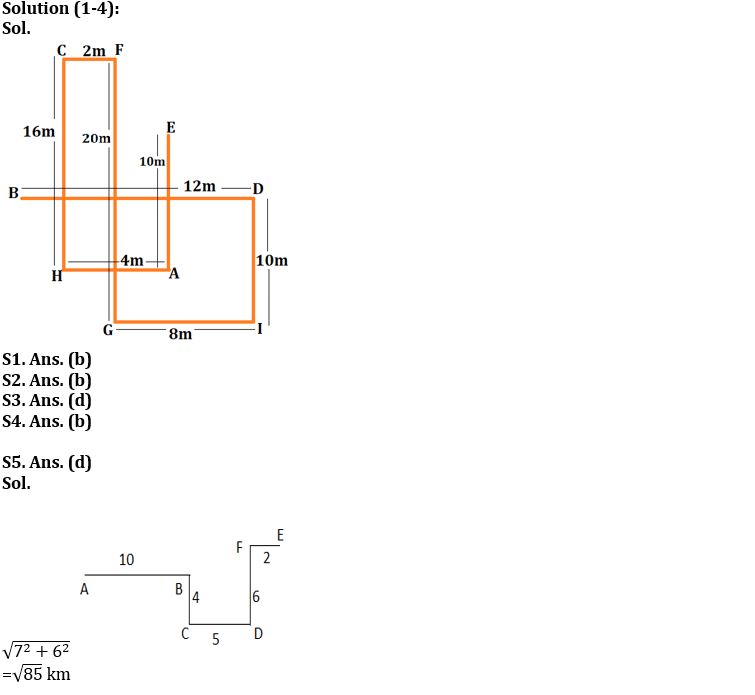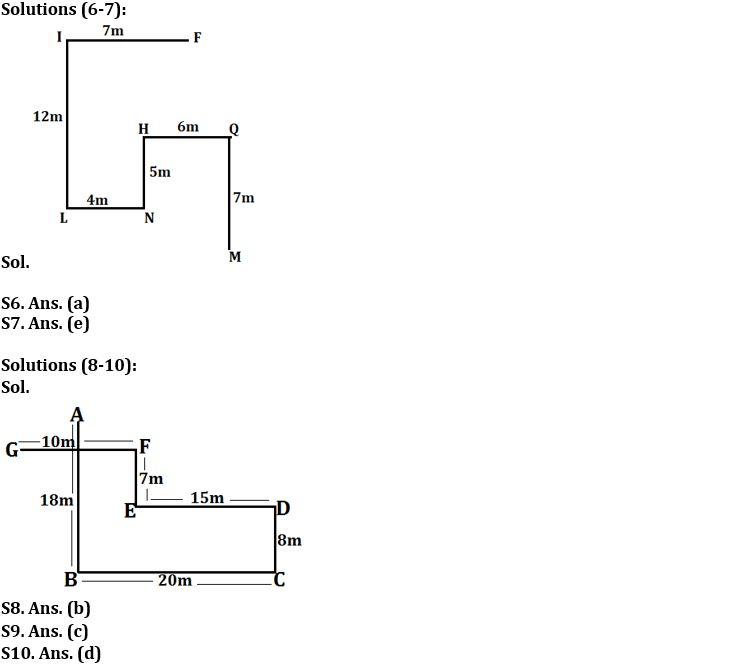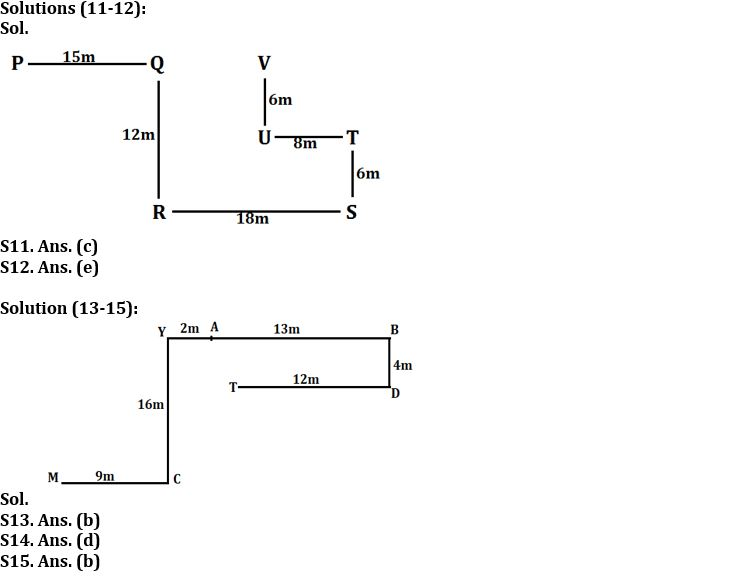#### Congratulations!Download Hindu Review of October 2021: Free PDFIncorrect details? Fill the form again here

Download Hindu Review of October 2021: Free PDF

Thank You, Your details have been submitted we will get back to you.
•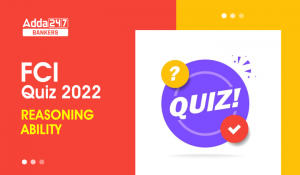Reasoning Ability Quiz For FCI Phase I 2...
•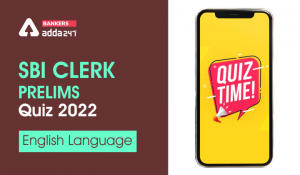English Quizzes For SBI Clerk Prelims 20...
•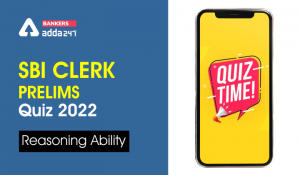Reasoning Ability Quiz For SBI Clerk Pre...
•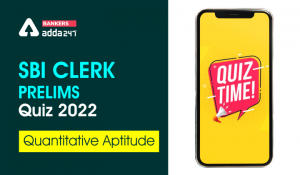Quantitative Aptitude Quiz For SBI Clerk...
•English Quizzes For SBI Clerk Prelims 20...
•Quantitative Aptitude Quiz For SBI Clerk...